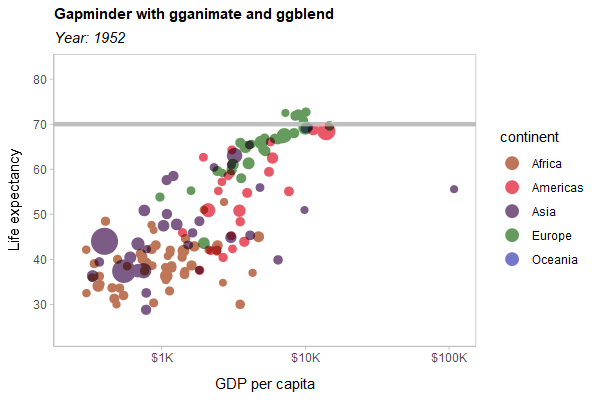ggblend adds support for blend modes (like "multiply", "overlay", etc) to ggplot2. It requires R >= 4.2, as blending and compositing support was added in that version of R.

## Installation

You can install the development version of ggblend using:

remotes::install_github("mjskay/ggblend")

## Blending within one geometry

We’ll construct a simple dataset with two semi-overlapping point clouds. We’ll have two versions of the dataset: one with all the "a" points listed first, and one with all the "b" points listed first.

library(ggplot2)
library(ggblend)
theme_set(theme_light())

set.seed(1234)
df_a = data.frame(x = rnorm(500, 0), y = rnorm(500, 1), set = "a")
df_b = data.frame(x = rnorm(500, 1), y = rnorm(500, 2), set = "b")

df_ab = rbind(df_a, df_b) |>
transform(order = "draw a then b")

df_ba = rbind(df_b, df_a) |>
transform(order = "draw b then a")

df = rbind(df_ab, df_ba)

A typical scatterplot of such data suffers from the problem that how many points appear to be in each group depends on the drawing order (a then b versus b then a):

df |>
ggplot(aes(x, y, color = set)) +
geom_point(size = 3) +
scale_color_brewer(palette = "Set2") +
facet_grid(~ order) +
ggtitle("Scatterplot without blending: draw order matters")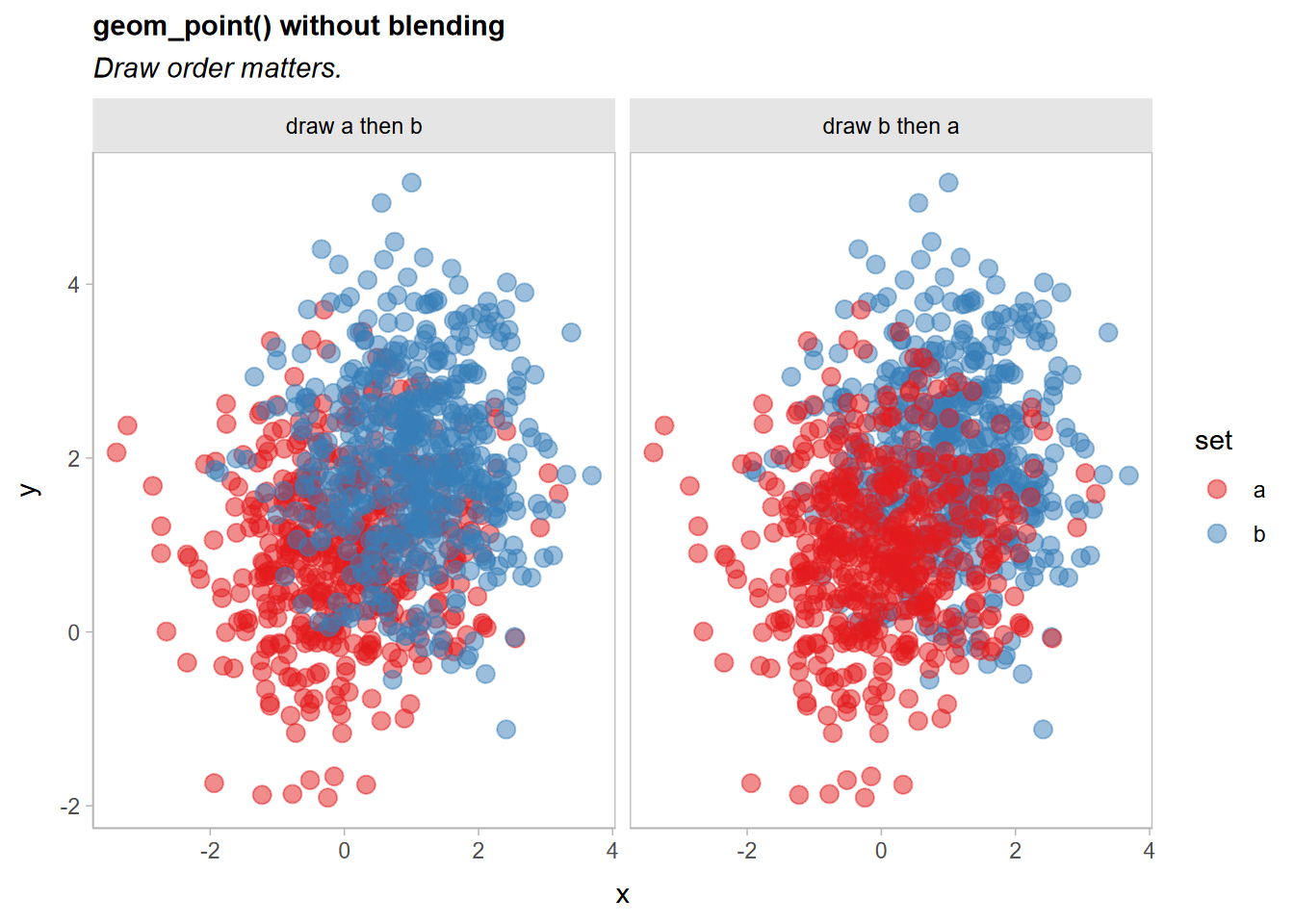A commutative blend mode, like "multiply" or "darken", is one potential solution that does not depend on drawing order. We can apply a blend() operation to geom_point() to achieve this. There three ways to do this:

• blend(geom_point(...), "darken") (normal function application)
• geom_point(...) |> blend("darken") (piping)
• geom_point(...) * blend("darken") (algebraic operations)

Function application and piping are equivalent. In this case, all three approaches are equivalent. As we will see later, the multiplication approach is useful when we want a shorthand for applying the same operation to multiple layers in a list without combining those layers first (in other words, multiplication of operations over layers is distributive in an algebraic sense).

df |>
ggplot(aes(x, y, color = set)) +
geom_point(size = 3) |> blend("darken") +
scale_color_brewer(palette = "Set2") +
facet_grid(~ order) +
ggtitle("Scatterplot with blend('darken'): draw order does not matter")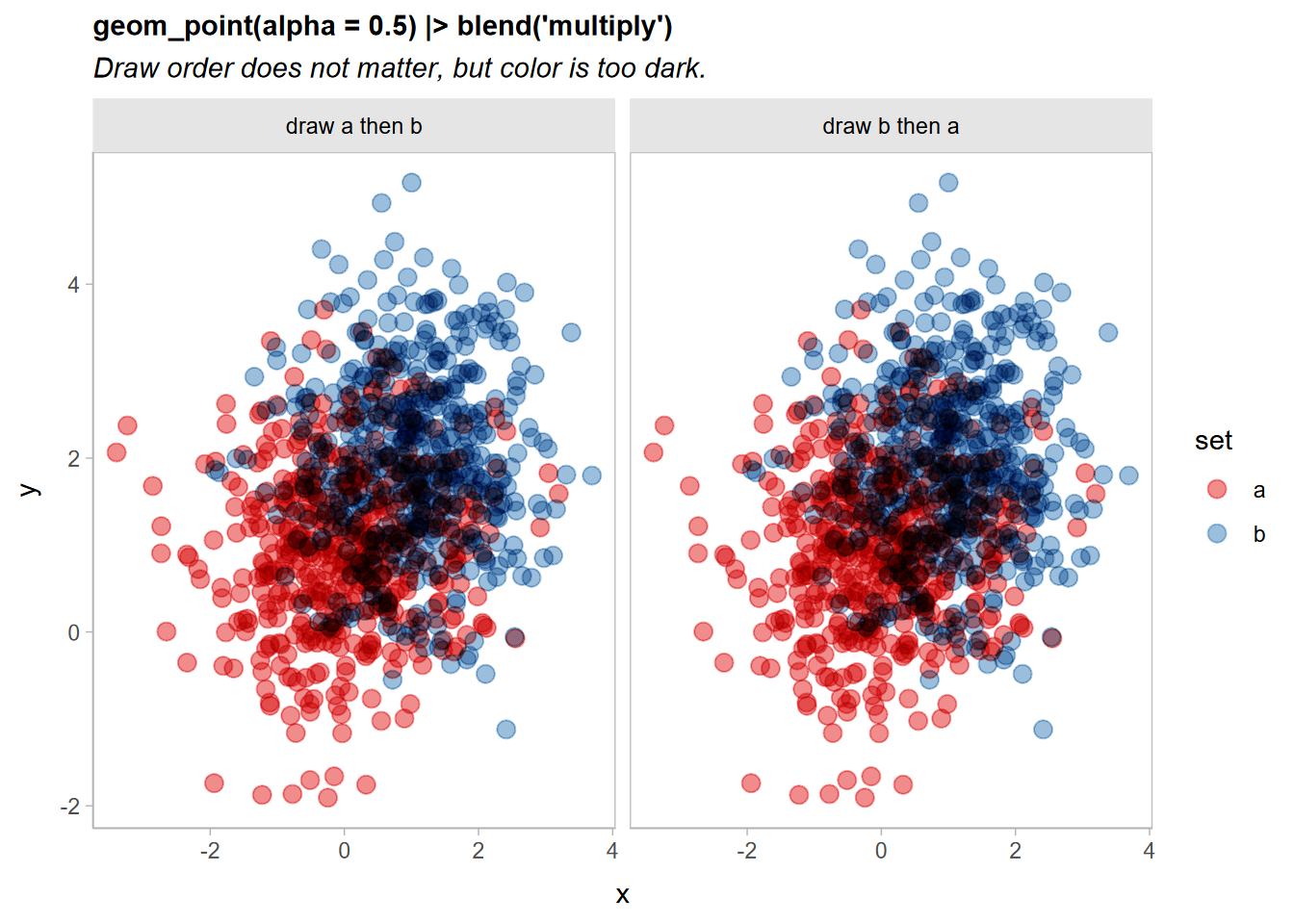Now the output is identical no matter the draw order.

## Alpha blending

One disadvantage of the "darken" blend mode is that it doesn’t show density within each group as well. We could use "multiply" instead, though then values can get quite dark:

df |>
ggplot(aes(x, y, color = set)) +
geom_point(size = 3) * blend("multiply") +
scale_color_brewer(palette = "Set2") +
facet_grid(~ order) +
ggtitle("Scatterplot with blend('multiply'): a little too dark")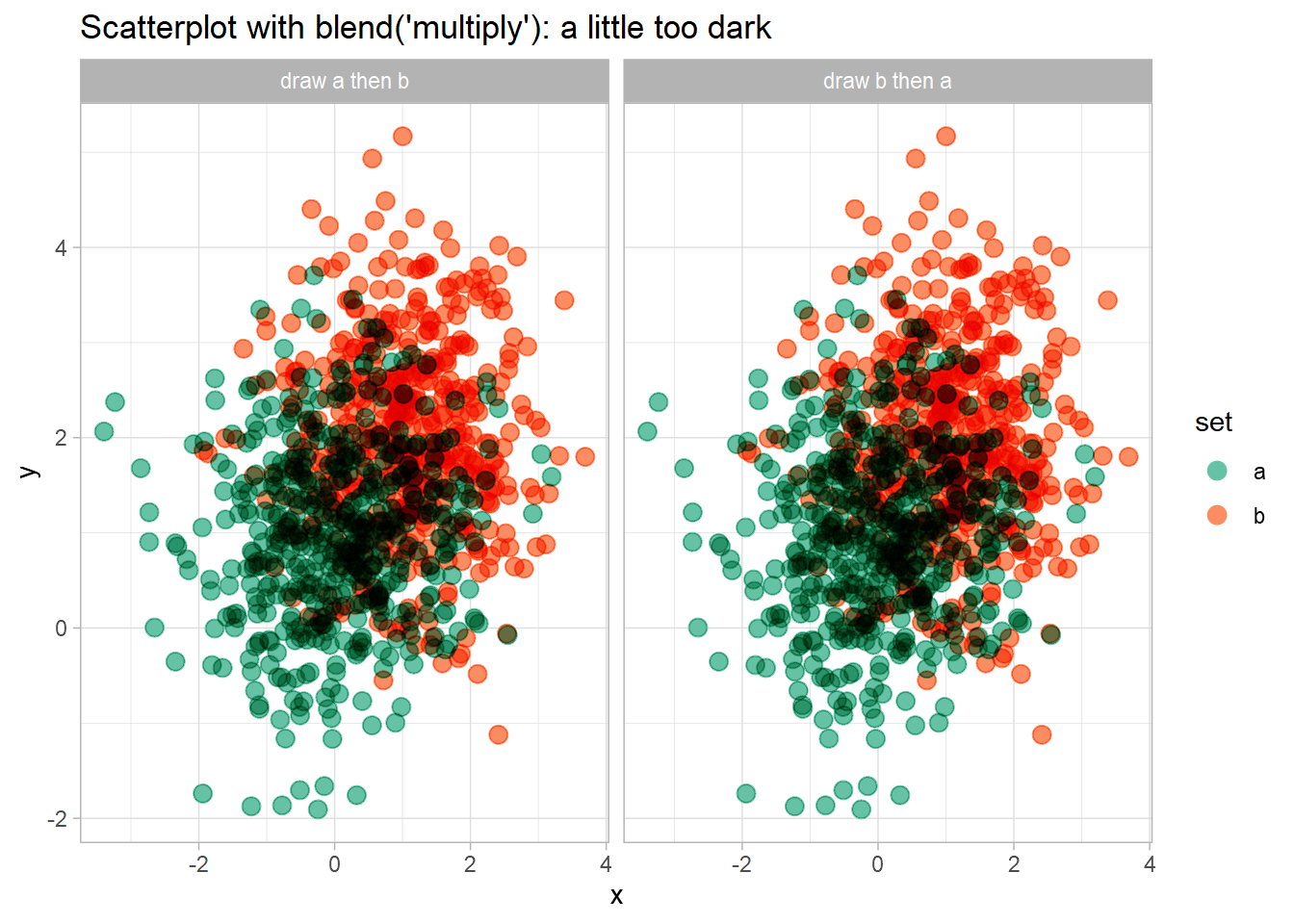Let’s try combining two blend modes to address this: we’ll use a "lighten" blend mode (which is also commutative, but which makes the overlapping regions lighter), and then draw the "multiply"-blended version on top at an alpha of less than 1:

df |>
ggplot(aes(x, y, color = set)) +
geom_point(size = 3) * blend("lighten") +
geom_point(size = 3) * blend("multiply", alpha = 0.65) +
scale_color_brewer(palette = "Set2") +
facet_grid(~ order) +
ggtitle("Scatterplot with blend('lighten') and blend('multiply', alpha = 0.65)")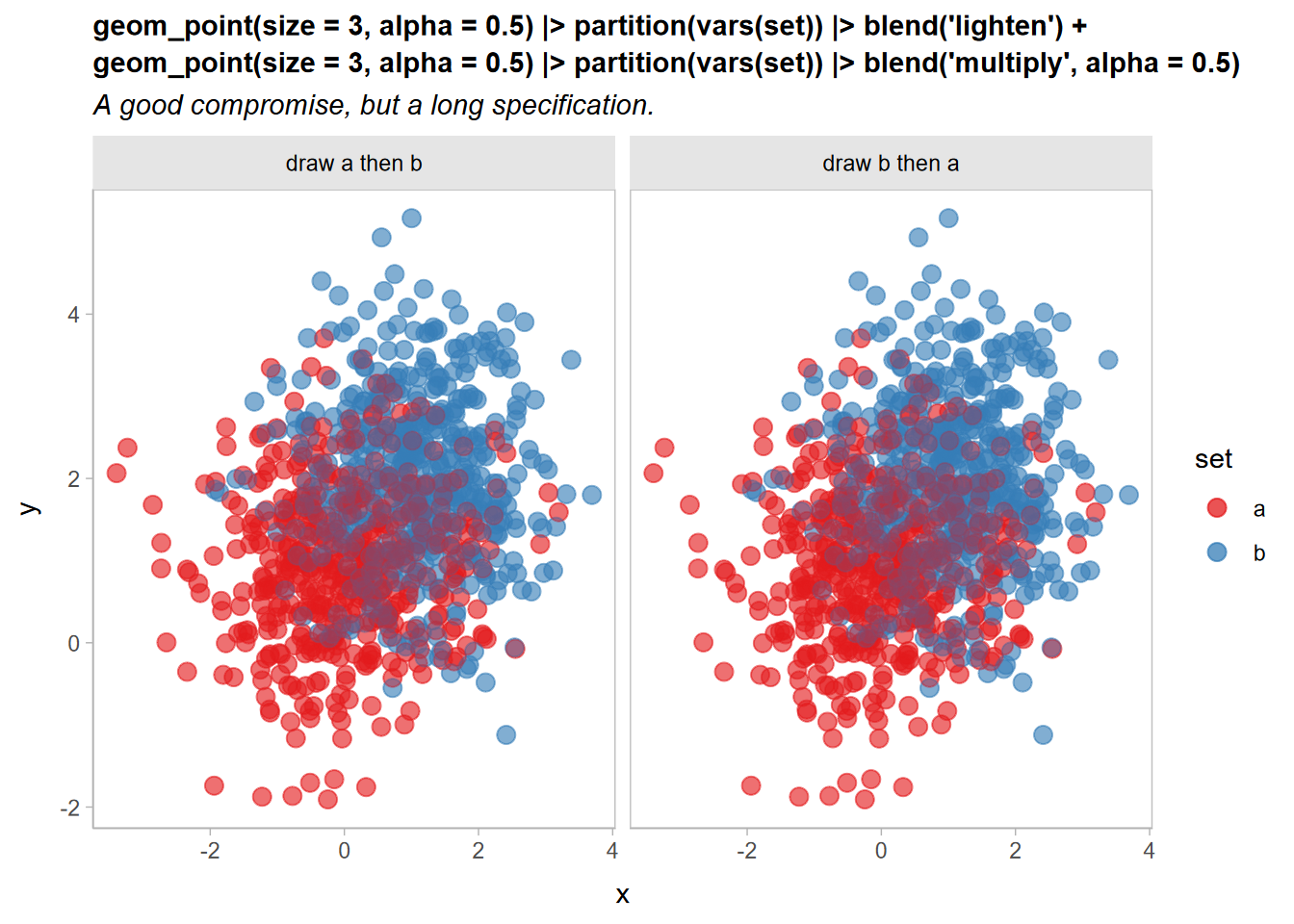Now it’s a little easier to see both overlap and density, and the output remains independent of draw order.

It is a little verbose to need to copy out a layer multiple times, as in:

geom_point(size = 3) * blend("lighten") +
geom_point(size = 3) * blend("multiply", alpha = 0.65)

Operations and layers in ggblend act as a small algebra. Operations and sums of operations can be multiplied by layers and lists of layers, and those operations are distributed over the layers (This is where * and |> differ: |> does not distribute operations like blend() over layers, which is useful if you want to use a blend to combine multiple layers together, rather than applying that blend to each layer individually).

For example, we can “factor out” the geom_point(size = 3) layer from the above expression, yielding this:

geom_point(size = 3) * (blend("lighten") + blend("multiply", alpha = 0.65))

Both expressions are equivalent. Thus we can rewrite the previous example like so:

df |>
ggplot(aes(x, y, color = set)) +
geom_point(size = 3) * (blend("lighten") + blend("multiply", alpha = 0.65)) +
scale_color_brewer(palette = "Set2") +
facet_grid(~ order) +
ggtitle("Scatterplot with blend('lighten') + blend('multiply', alpha = 0.65)")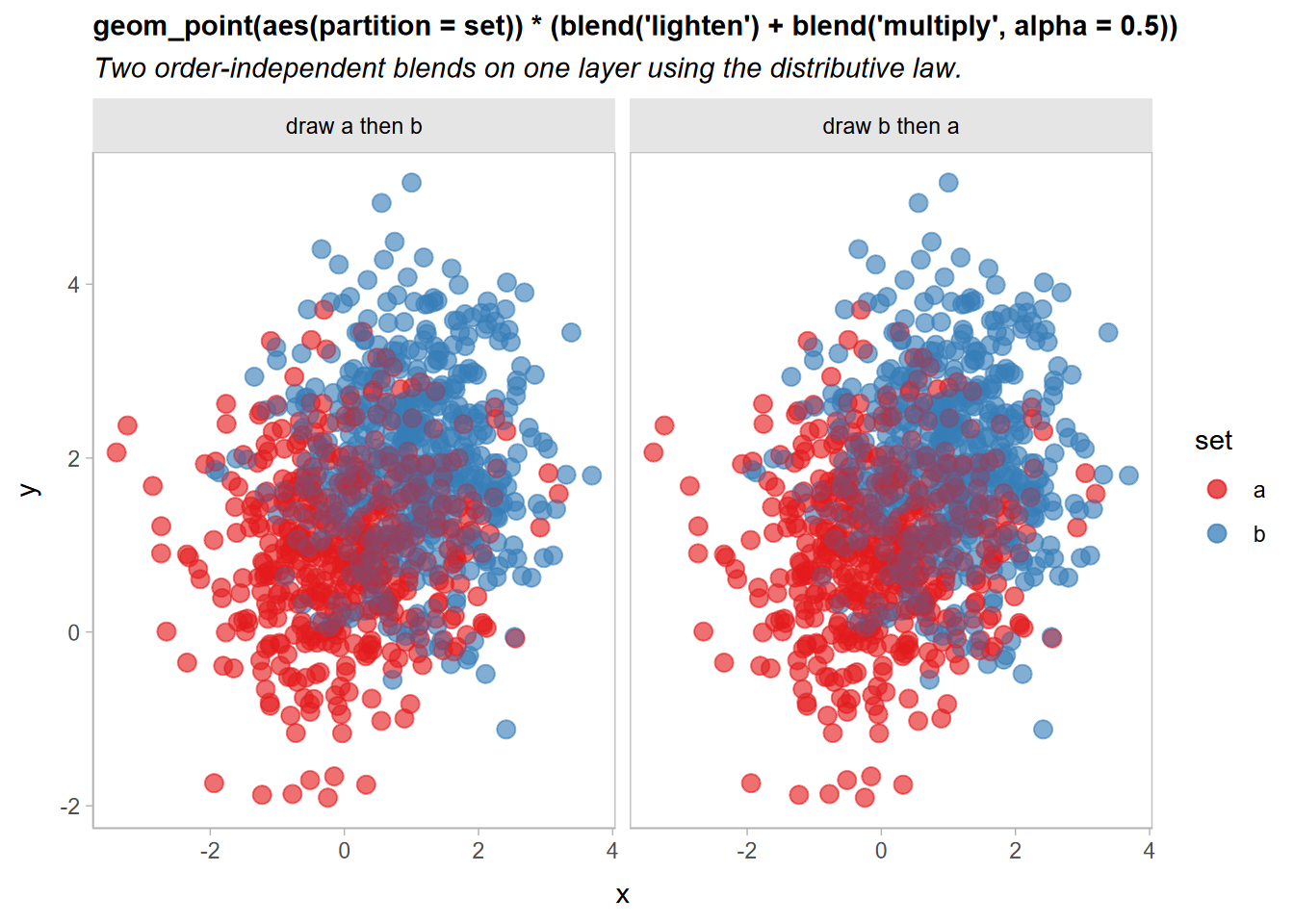## Blending multiple geometries

We can also blend geometries together by passing a list of geometries to blend(). These lists can include already-blended geometries:

df |>
ggplot(aes(x, y, color = set)) +
list(
geom_point(size = 3) * (blend("lighten") + blend("multiply", alpha = 0.65)),
geom_vline(xintercept = 0, color = "gray75", size = 1.5),
geom_hline(yintercept = 0, color = "gray75", size = 1.5)
) |> blend("hard.light") +
scale_color_brewer(palette = "Set2") +
facet_grid(~ order) +
labs(title = "Blending multiple geometries together")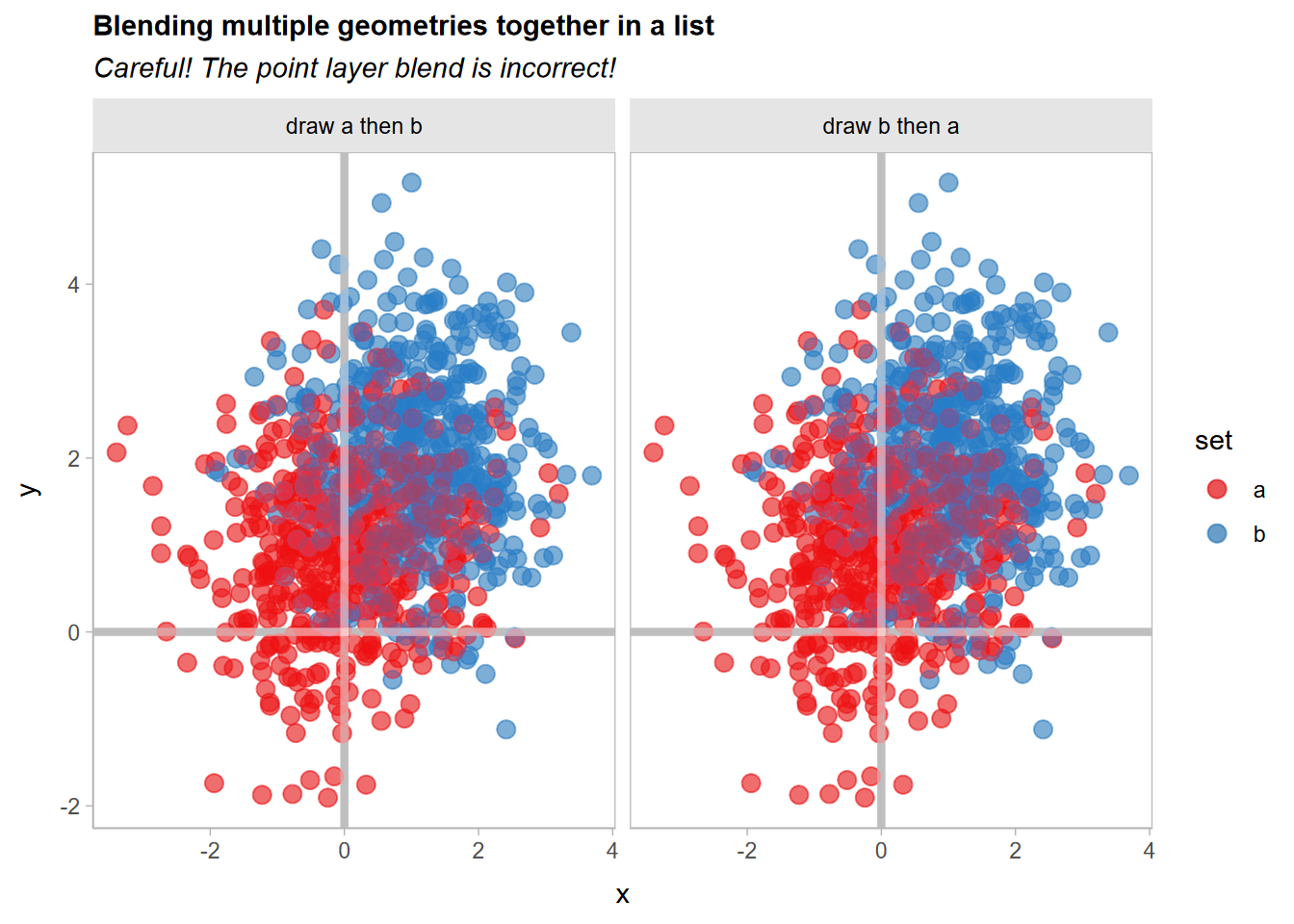Whoops!! If you look closely, the blending of the geom_point() layers appears to have changed. Recall that this expression:

geom_point(size = 3) * (blend("lighten") + blend("multiply", alpha = 0.65))

Is equivalent to specifying two separate layers, one with blend("lighten") and the other with blend("multiply", alpha = 0.65)). Thus, when you apply |> blend("hard.light") to the list() of layers, it will use a hard light blend mode to blend these two layers together, when previously they would be blended using the normal (or "over") blend mode.

We can gain back the original appearance by blending these two layers together with |> blend() prior to applying the hard light blend:

df |>
ggplot(aes(x, y, color = set)) +
list(
geom_point(size = 3) * (blend("lighten") + blend("multiply", alpha = 0.65)) |> blend(),
geom_vline(xintercept = 0, color = "gray75", size = 1.5),
geom_hline(yintercept = 0, color = "gray75", size = 1.5)
) |> blend("hard.light") +
scale_color_brewer(palette = "Set2") +
facet_grid(~ order) +
labs(title = "Blending multiple geometries together")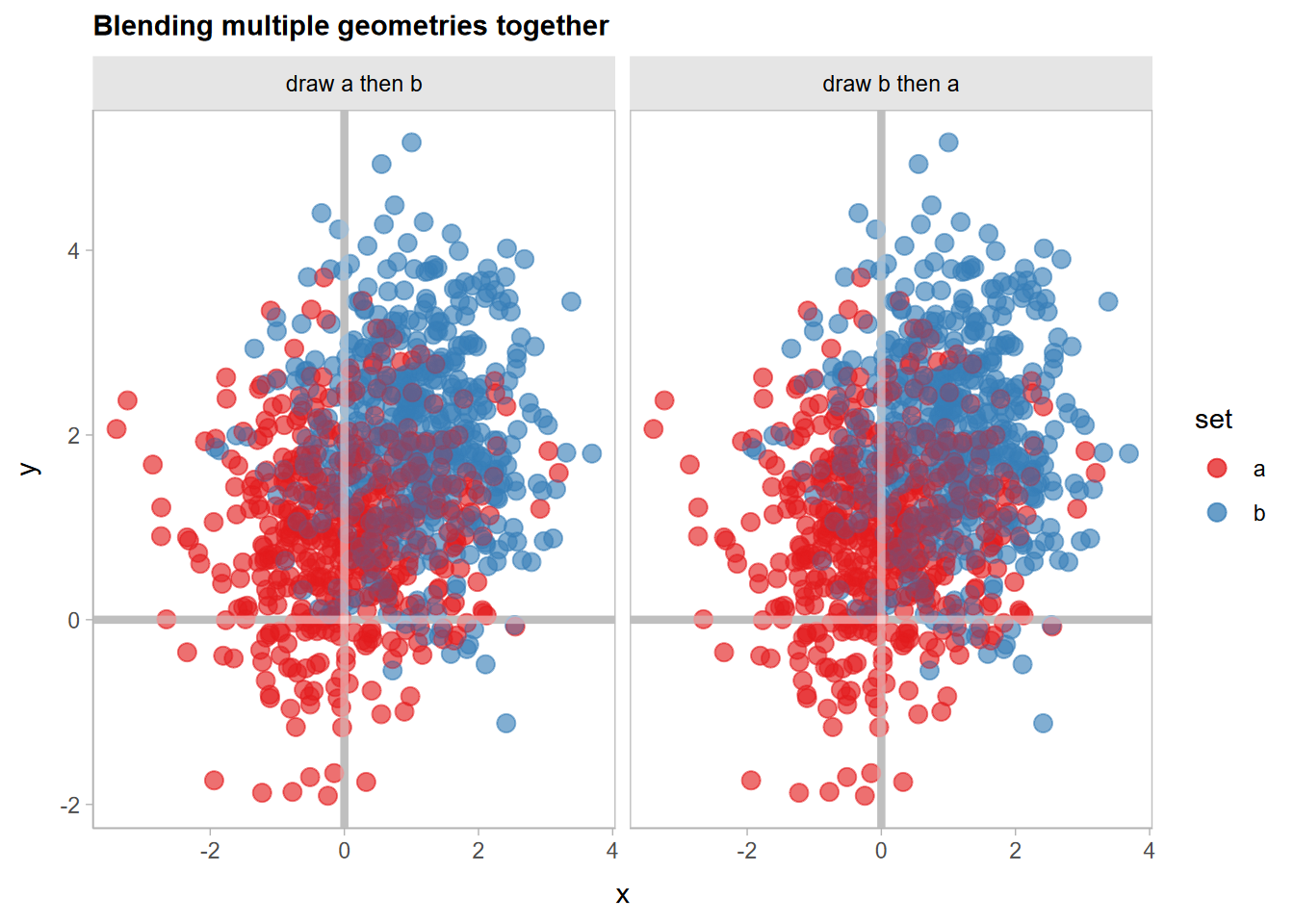## Blending groups within one geometry

Sometimes it’s useful to have finer-grained control of blending within a given geometry. Here, we’ll show how to use blend() with stat_lineribbon() from ggdist to create overlapping gradient ribbons depicting uncertainty.

We’ll fit a model:

m_mpg = lm(mpg ~ hp * cyl, data = mtcars)

And generate some confidence distributions for the mean using distributional:

grid = unique(mtcars[ c("cyl", "hp")])

predictions = grid |>
cbind(predict(m_mpg, newdata = grid, se.fit = TRUE)) |>
transform(mu_hat = distributional::dist_student_t(df = df, mu = fit, sigma = se.fit))

predictions
##                     cyl  hp      fit    se.fit df residual.scale
## Mazda RX4             6 110 20.28825 0.7984429 28       2.973694
## Datsun 710            4  93 25.74371 0.8818612 28       2.973694
## Hornet Sportabout     8 175 15.56144 0.8638133 28       2.973694
## Valiant               6 105 20.54952 0.8045354 28       2.973694
## Duster 360            8 245 14.66678 0.9773475 28       2.973694
## Merc 240D             4  62 28.58736 1.2184598 28       2.973694
## Merc 230              4  95 25.56025 0.9024699 28       2.973694
## Merc 280              6 123 19.60892 0.8423540 28       2.973694
## Merc 450SE            8 180 15.49754 0.8332276 28       2.973694
## Cadillac Fleetwood    8 205 15.17801 0.7674501 28       2.973694
## Lincoln Continental   8 215 15.05021 0.7866649 28       2.973694
## Chrysler Imperial     8 230 14.85849 0.8606705 28       2.973694
## Fiat 128              4  66 28.22044 1.1218796 28       2.973694
## Honda Civic           4  52 29.50466 1.4914670 28       2.973694
## Toyota Corolla        4  65 28.31217 1.1451536 28       2.973694
## Toyota Corona         4  97 25.37679 0.9280143 28       2.973694
## Dodge Challenger      8 150 15.88096 1.0770037 28       2.973694
## Porsche 914-2         4  91 25.92718 0.8665404 28       2.973694
## Lotus Europa          4 113 23.90910 1.2628426 28       2.973694
## Ford Pantera L        8 264 14.42394 1.1660619 28       2.973694
## Ferrari Dino          6 175 16.89163 1.5508852 28       2.973694
## Maserati Bora         8 335 13.51650 2.0458075 28       2.973694
## Volvo 142E            4 109 24.27603 1.1625257 28       2.973694
##                                         mu_hat
## Mazda RX4           t(28, 20.28825, 0.7984429)
## Datsun 710          t(28, 25.74371, 0.8818612)
## Hornet Sportabout   t(28, 15.56144, 0.8638133)
## Valiant             t(28, 20.54952, 0.8045354)
## Duster 360          t(28, 14.66678, 0.9773475)
## Merc 240D             t(28, 28.58736, 1.21846)
## Merc 230            t(28, 25.56025, 0.9024699)
## Merc 280             t(28, 19.60892, 0.842354)
## Merc 450SE          t(28, 15.49754, 0.8332276)
## Cadillac Fleetwood  t(28, 15.17801, 0.7674501)
## Lincoln Continental t(28, 15.05021, 0.7866649)
## Chrysler Imperial   t(28, 14.85849, 0.8606705)
## Fiat 128              t(28, 28.22044, 1.12188)
## Honda Civic          t(28, 29.50466, 1.491467)
## Toyota Corolla       t(28, 28.31217, 1.145154)
## Toyota Corona       t(28, 25.37679, 0.9280143)
## Dodge Challenger     t(28, 15.88096, 1.077004)
## Porsche 914-2       t(28, 25.92718, 0.8665404)
## Lotus Europa          t(28, 23.9091, 1.262843)
## Ford Pantera L       t(28, 14.42394, 1.166062)
## Ferrari Dino         t(28, 16.89163, 1.550885)
## Maserati Bora         t(28, 13.5165, 2.045807)
## Volvo 142E           t(28, 24.27603, 1.162526)

A basic plot based on examples in vignette("freq-uncertainty-vis", package = "ggdist") and vignette("lineribbon", package = "ggdist") may have issues when lineribbons overlap:

predictions |>
ggplot(aes(x = hp, fill = ordered(cyl), color = ordered(cyl))) +
ggdist::stat_lineribbon(
aes(ydist = mu_hat, fill_ramp = stat(.width)),
.width = ppoints(40)
) +
geom_point(aes(y = mpg), data = mtcars) +
scale_fill_brewer(palette = "Set2") +
scale_color_brewer(palette = "Dark2") +
ggdist::scale_fill_ramp_continuous(range = c(1, 0)) +
labs(
title = "Overlapping lineribbons without blending",
color = "cyl", fill = "cyl", y = "mpg"
) +
ggdist::theme_ggdist()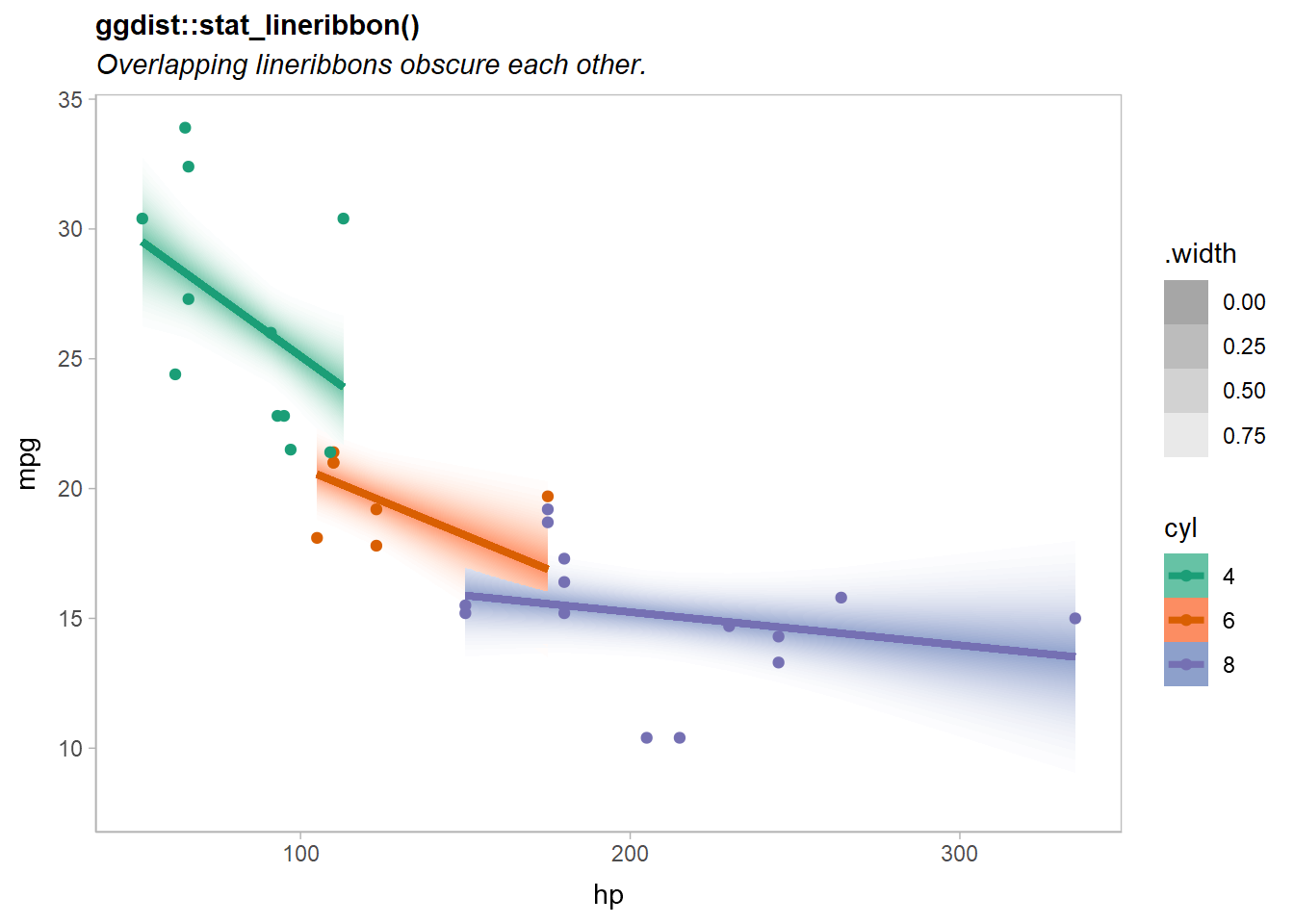Notice the overlap of the orange (cyl = 6) and purple (cyl = 8) lines.

If we add a partition = cyl aesthetic mapping, we can blend the geometries for the different levels of cyl together with a blend() call around ggdist::stat_lineribbon().

There are many ways we could add the partition to the plot:

1. Add partition = cyl to the existing aes(...) call. However, this leaves the partitioning information far from the call to blend(), so the relationship between them is less clear.
2. Add aes(partition = cyl) to the stat_lineribbon(...) call. This is a more localized change (better!), but will raise a warning if stat_lineribbon() itself does not recognized the partition aesthetic.
3. Add |> adjust(aes(partition = cyl)) after stat_lineribbon(...) to add the partition aesthetic to it (this will bypass the warning).
4. Add |> partition(vars(cyl)) after stat_lineribbon(...) to add the partition aesthetic. This is an alias for the adjust() approach that is intended to be clearer. It takes a specification for a partition that is similar to facet_wrap(): either a one-sided formula or a call to vars().

Let’s try the fourth approach:

predictions |>
ggplot(aes(x = hp, fill = ordered(cyl), color = ordered(cyl))) +
ggdist::stat_lineribbon(
aes(ydist = mu_hat, fill_ramp = stat(.width)),
.width = ppoints(40)
) |> partition(vars(cyl)) |> blend("multiply") +
geom_point(aes(y = mpg), data = mtcars) +
scale_fill_brewer(palette = "Set2") +
scale_color_brewer(palette = "Dark2") +
ggdist::scale_fill_ramp_continuous(range = c(1, 0)) +
labs(
title = "Overlapping lineribbons with aes(partition = cyl) and blend('multiply')",
color = "cyl", fill = "cyl", y = "mpg"
) +
ggdist::theme_ggdist()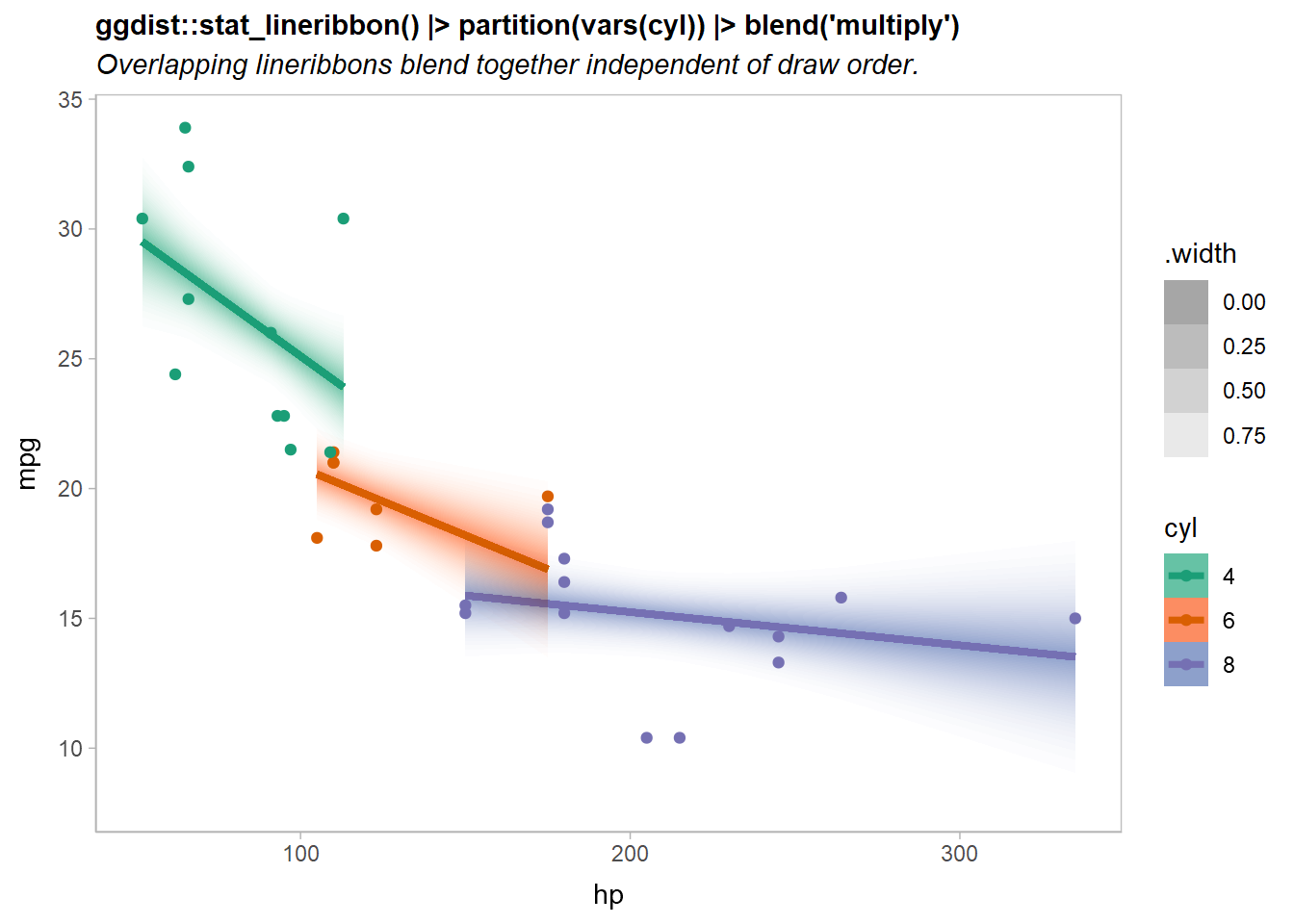Now the overlapping ribbons are blended together.

## Compatibility with other packages

In theory ggblend should be compatible with other packages, though in more complex cases (blending lists of geoms or using the partition aesthetic) it is possible it may fail, as these features are a bit more hackish.

As a hard test, here is all three features applied to a modified version of the Gapminder example used in the gganimate documentation:

library(gganimate)
library(gapminder)

p = ggplot(gapminder, aes(gdpPercap, lifeExp, size = pop, color = continent, partition = continent)) +
list(
geom_point(show.legend = c(size = FALSE)) |> blend("multiply"),
geom_hline(yintercept = 70, size = 2.5, color = "gray75")
) |> blend("hard.light") +
scale_color_manual(values = continent_colors) +
scale_size(range = c(2, 12)) +
scale_x_log10() +
labs(
title = 'Gapminder with gganimate and ggblend',
subtitle = 'Year: {frame_time}',
x = 'GDP per capita',
y = 'life expectancy'
) +
transition_time(year) +
ease_aes('linear')

animate(p, type = "cairo")`